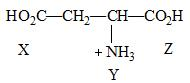Courses

# The pKa values for the three ionizable groups, X, Y and Z of glutamic acid are 4.3, 9.7 and 2.2 respectively:The isoelectric point for the amino acid is:Correct answer is between '3.1,3.3'. Can you explain this answer? Related Test: IIT JAM Chemistry Mock Test 1

## Chemistry Question

By Shoaib Ali · Feb 07, 2020 ·Chemistry
Prerna Sheoran answered Jul 11, 2019
To find isoelectric point for an amino acid,first find out its nature i.e.is it acting like a base or an acid.
For acidic amino acid isoelectric point will be average of two highest pka values and for basic amino acid isoelectric point will be average of lowest two pka values,here amino acid has accepted H+ ion,so it is acting as basic amino acid so isoelectric point will be average of lowest two pKa values.here it will be average of 4.3 and 2.2 i.e.(4.3+2.2)/2=3.25